# Combinations and Permutations

## Combinations:

Sometimes, we want to count all of the possible ways that a single set of objects can be selected – without regard to the order in which they are selected.

• In general, n objects can be arranged in n(n – 1)(n – 2) … (3)(2)(1) ways. This product is represented by the symbol n!, which is called n factorial. (By convention, 0! = 1.)
• combination is a selection of all or part of a set of objects, without regard to the order in which they were selected. This means that XYZ is considered the same combination as ZYX.
• The number of combinations of n objects taken r at a time is denoted by nCr.

Rule 1. The number of combinations of n objects taken r at a time is

nCr = n(n – 1)(n – 2) … (n – r + 1)/r! = n! / r!(n – r)!

Combinations are easy in which order doesn’t matter. You can mix it up and it looks the same. e.g. How many ways can I give 3 pencil to 8 children?

In this case, the order we pick student doesn’t matter. If I give a pencil to Rashid, Ali and Mahmood, it’s the same as giving to Mahmood, Ali and Rashid.

For a moment, let’s think out how many ways we can rearrange these three students.

we have 3 choices for the first student, 2 for the second, and only 1 for the last. So we have 3 · 2 · 1 ways to re-arrange 3 people.

Wait a while………………. this is looking a bit like a permutation! You tricked me!

Indeed I did. If you have N number of students and you want to know how many arrangements there are for all of them, it’s just N factorial or N!

So, if we have 3 pencils to give away, there are 3! or 6 variations for every choice we pick. If we want to see how many combinations we have, we just create all the permutations and divide by all the redundancies. In our case, we get 336 permutations (from above), and we divide by the 6 redundancies for each permutation and get 336/6 = 56.

The general formula is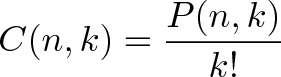$\displaystyle{C(n,k) = \frac{P(n,k)}{k!}}$

which means “Find all the ways to pick k people from n, and divide by the k! variants”. Writing this out, we get our combination formula, or the number of ways to combine k items from a set of n:$\displaystyle{C(n,k) = \frac{n!}{(n-k)!k!}}$

Examples:

• Combination: Picking a team of 3 players from a group of 10.

C(10,3) = 10!/(7! · 3!) = 10 · 9 · 8 / (3 · 2 · 1) = 120.

• Choosing 3 sweets from a menu of 10.

C(10,3) = 120.

## Permutations:

Permutations is the all possible ways of doing something but order matter.  Let’s say we have 8 players:

1. Alexander
2. Joshi
3. Khalid
4. Rizwan
5. Ali
6. Pasha
7. Pervaiz
8. Shahid

How many ways can we award a 1st, 2nd and 3rd place prize among eight contestants?

(Gold / Silver / Bronze)

A, B, C, D, E, F, G, H = 8 choices

B, C, D, E, F, G, H = 7 choices

C, D, E, F, G, H = 6 choices

In permutations since the order we hand out these medals matters. Here’s how it breaks down:

• Gold medal: 8 choices: A B C D E F G H (Clever how I made the names match up with letters, eh?).
• Let’s say A wins the Gold.
• Silver medal: 7 choices: B C D E F G H. Let’s say B wins the silver.
• Bronze medal: 6 choices: C D E F G H. Let’s say… C wins the bronze.

We picked certain players to win but here, the details does not matter.

we had 8 choices at first, then 7, then 6. The total number of options was 8 · 7 · 6 = 336.

Let’s look at the details. We had to order 3 players out of 8. To do this, we started with all options (8) then took them away one at a time (7, then 6) until we ran out of medals. We know the factorial is: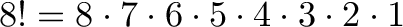$\displaystyle{ 8! = 8 \cdot 7 \cdot 6 \cdot 5 \cdot 4 \cdot 3 \cdot 2 \cdot 1 }$

But unfortunately, does too much! We only want 8 · 7 · 6. How can we “stop” the factorial at 5?, So, try to find out this one. This is where permutations get cool: notice how we want to get rid of 5 · 4 · 3 · 2 · 1. What’s another name for this? 5 factorial! So, if we do 8!/5! we get: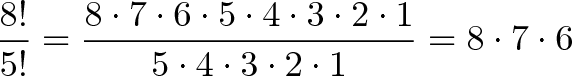$\displaystyle{\frac{8!}{5!} = \frac{8 \cdot 7 \cdot 6 \cdot 5 \cdot 4 \cdot 3 \cdot 2 \cdot 1}{5 \cdot 4 \cdot 3 \cdot 2 \cdot 1} = 8 \cdot 7 \cdot 6}$

And why did we use the number 5? This is because, it was left over after we picked 3 medals from 8. So, a better way to write this would be: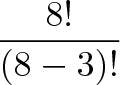$\displaystyle{\frac{8!}{(8-3)!}}$

where 8!/(8-3)! is just a fancy way of saying “Use the first 3 numbers of 8!”. If we have n items total and want to pick k in a certain order, we get:$\displaystyle{\frac{n!}{(n-k)!}}$

And this is the fancy permutation formula: You have n items and want to find the number of ways k items can be ordered: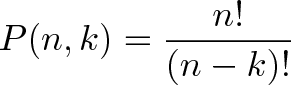$\displaystyle{P(n,k) = \frac{n!}{(n-k)!}}$

Examples:

• Picking a President, Vice President and General Secretary from a group of 10.

P(10,3) = 10!/7! = 10 · 9 · 8 = 720.

• Listing your 3 favorite sweets, in order, from a menu of 10.

P(10,3) = 720.

nc